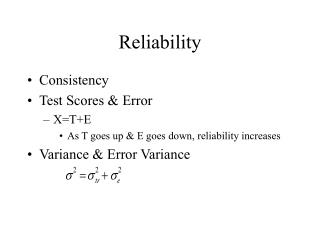Download PresentationReliability

# Reliability - PowerPoint PPT PresentationDownload Presentation## Reliability

- - - - - - - - - - - - - - - - - - - - - - - - - - - E N D - - - - - - - - - - - - - - - - - - - - - - - - - - -
##### Presentation Transcript

1. Reliability • Consistency • Test Scores & Error • X=T+E • As T goes up & E goes down, reliability increases • Variance & Error Variance

2. Sources of Error • Test Construction/Content • Sampling; finite number of questions • Poorly written questions • Test Administration • Error related to the test taker • Error related to the test environment • Error related to the examiner

3. Sources of Error (cont.) • Test scoring & interpretation • Objective v. subjective • scoring rubrics

4. Parallel Tests • Theoretical underpinning of reliability • Similar content • Same true score & same error variance • Theoretical, not produced in reality • Not to be confused with “alternate forms” • Reliability can be defined as the correlation between 2 parallel tests

5. Types of Reliability • Reliability over time • Internal consistency/reliability • Inter-rater reliability

6. Reliability over time • Test-retest reliability • Obtained by correlating pairs of scores from the same sample on two different administrations of the same test • Error related to passage of time & intervening factors • Alternate-Form (Immediate & Delayed) • Error related to time & content

7. Internal Consistency • Split-half • Divide the test into two equivalent halves • Odd-even • Randomly assign items • Divide by equivalency of items • Calculate r between 2 halves • Correct with Spearman-Brown • Allows estimation of reliability of test that has been shortened or lengthened

8. Internal Consistency (cont.) • Inter-item consistency • Index of homogeneity of test; degree to which all items measure same construct • Desirable: aids in interpretation of test (as opposed to homogeneity of groups)

9. Internal Consistency (cont.) • Kuder-Richardson formulas • KR-20: statistic of choice for determining reliability of tests with dichotomous items (right-wrong) • KR-21: can be used if assumption that all items are of similar difficulty

10. Internal Consistency (cont.) • Cronbach’s coefficient alpha • Function of all items on test & the total test score • Each item conceptualized as a test • 36-item test, 36-parallel tests • In addition to use with dichotomous tests can be used with tests containing nondichotomous items, e.g., opinion, tests which allow partial credit

11. Inter-rater reliability • How well do 2 raters/judges agree? • Correlation between scores from 2 raters • Percentage of agreement; percentage of intervals where both raters agreed behavior occurred • Kappa

12. Factors influencing reliability • Length of test • Longer tests increase percentage of domain that can be sampled • Point of diminishing returns • Homogeneity of items • Measure same construct; easier to interpret • Dynamic or static characteristics

13. Factors influencing reliability (cont.) • Homogeneity of sample • Restriction of range • If sample is homogenous then any observed variance must be error • Power v. Speed tests • Speed use test-retest; alternate forms; split half from 2 separately timed half tests • Internal consistency not applicable • Speed tests easy; internal consistency inflates reliability

14. Reliability of Individual Scores • How much error is in an individual score? • How much confidence do we have in a particular score? • Standard Error of Measurement • Extent to which one individual’s scores vary over tests that are presumed to be parallel • Assume error is distributed “normally” • Where is the individual’s “true” score?

15. Standard Error of Measurement

16. SEM (cont.) • Odds are 68% that “true” score falls within plus or minus 1 SEM. • Odds are __% that “true” score falls within plus or minus 2 (1.96) SEM. • Odds are __% that “true” score falls within plus or minus 3 SEM. • WHAT IS THE RELATIONSHIP BETWEEN RELIABILITY & SEM?

17. Standard Error of the Difference of Two Scores • Compare test takers performance on two different tests • Compare two test takers on the same test • Compare two test takers on two different tests

18. Standard Error of the Difference

19. Standard Error of the Difference • Set confidence intervals for difference scores • Difference scores contain error from both of the comparison measures. • Difference scores are less reliable than scores from individual tests.

20. Test-retest reliability:Social Interaction Self-Statement • r+1+2 = .99 • r-1-2 = .99 • r+1-1 = -.45 • r+1-2 = -.55 • r+2-1 = -.47 • r+2-2 = -.56